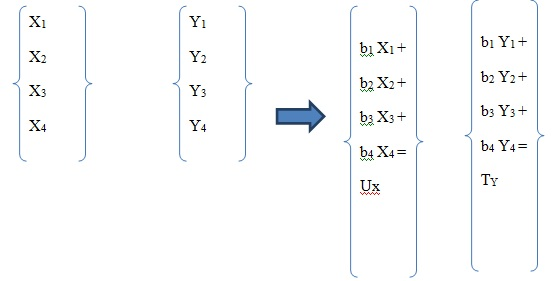# Performing Canonical Correlation Analysis (CCA)

Until recently, Karl Pearson Correlation analysis was one of the most popular methods to measure linear association between two or more than two variables in a data set. For example, establishing the Karl Pearson Correlation between X variable and Y variable, where both variables belong to a single data set. Canonical Correlation Analysis (CCA), on the other hand, helps measure the correlation among variables which are in different datasets.

For example, below figure shows two datasets:Figure 1 Graph showing canonical correlation from two different data sets

Here, Canonical Correlation Analysis helps to estimate the possible association of different variables (like, age, sex and diet) with variables of other dataset (like heartbeat rate, hemoglobin and blood pressure).  CCA is a well-known multivariate analysis method for quantifying the correlation between two sets of multidimensional variables.

## How Canonical Correlation Analysis works?

As discussed above, CCA works with two different data sets. However, instead of taking correlation of each variable with other variable, it has a different technique. It takes a correlation analysis among the linear combinations of two data sets.Figure 2: Procedure of canonical correlation analysis taking the linear combination from data set X and Y

For instance, there are two data sets X and Y. Canonical correlations works with linear combinations of X’s variables and Y’s variables using different weights “bi”. Thereafter, a correlation between linear combination is formed with “UX” and “TY”.

## Important Assumptions for Canonical Correlation

1. One of the key assumptions that Canonical Correlation Analysis is based on is that the variables in the population should have Multivariate Normal or Gaussian Distribution from which the sample was taken.
2. Canonical Correlation Analysis requires a large sample size same as the multivariate regression to generate a robust model.
3. Canonical correlations analysis cannot be performed if multicollinearity is found among one or more variable sets. In other words, none of the variables should have correlations equal to 1 among each other.

## Example for Canonical Correlation

To show the strength of association between five aptitude tests and three tests on math, reading and writing, two data sets one as standard tests (Maths, Reading and writing) and other data set as aptitude Tests (Apt1, Apt2, Apt3, Apt4 and Apt5).

Table 1: The first table reports the canonical correlation coefficients and the eigenvalues of the canonical roots. The first canonical correlation coefficient is .65723 with an explained variance of the correlation of 74.26% and an eigenvalue of 0.76042. This indicates that the hypothesis is correct as the three test scores and all five aptitude test scores are positively related.

Table 2: The second table runs and shows the significance test results for all three canonical roots at the significance level p-value<0.05 individually (Roots 1 To 3, 2 To 3, and 3 To 3). ‘Root 1 To 3’ shows all the canonical roots included. ‘Roots 2 To 3’ considers only the last two canonical roots, while ‘Roots 3 To 3’ has just the last canonical root. In this example none of the root is significant at p-value < .05.Figure 4: Results from canonical correlation

## Application of Canonical Correlation Analysis

Where multi-data sets are available, CCA is applicable. For example,

• A credit card company can apply CCA to find out the association between bank account type (Current, Savings, or Fixed Deposits) with credit cards taken.
• A healthcare research centre can apply CCA to test the association between predictors of any disease based on medical history of patients.
• Insurance companies use CCA to test association between type of insurance policies taken like life insurance, health insurance etc. and characteristics of individuals like income, age, gender, medical background.
• Marketers use CCA in examining association between customers’ demographic factors and preferences for different products.

Softwares that support CCA with multiple independent variables are R, SAS, MATLAB, STATA and SPSS. However, SPSS does not include a separate command for CCA; in that case, it is carried out using Syntax.

### Priya Chetty

Partner at Project Guru
Priya is a master in business administration with majors in marketing and finance. She is fluent with data modelling, time series analysis, various regression models, forecasting and interpretation of the data. She has assisted data scientists, corporates, scholars in the field of finance, banking, economics and marketing.

### Related articles

• How to conduct generalized least squares test? In statistics, Generalized Least Squares (GLS) is one of the most popular methods for estimating unknown coefficients of a linear regression model when the independent variable is correlating with the residuals.
• How to detect outliers in a dataset? Outliers are those data points which are distant from the other observations in the data set. They can be either because of the variability in the data set or due to measurement errors.
• How to perform nonlinear regression? Regression analysis is a statistical tool to study the relationship between variables. These variables are the outcome variable and one or more exposure variables. In other words, regression analysis is an equation which predicts a response from the value of a certain predictor.
• How to apply logistic regression in a case? Machine learning involves solutions to predict scenarios based on past data. Logistic regression offers probability functions based on inputs and their corresponding output.
• How to conduct path analysis? Path analysis is a graphical representation of multiple regression models. In this analysis, the graphs represent the relationship between dependent and independent variables with the help of square and arrows.
Discussions

1.thaya
2.thaya